Navigation C API Pages Python bindings Applications

## Python Filters module

The python binding maps mostly to the C API with the gp_filter_ prefix stripped.

The filter functions could be called directly as `filters.Foo(img, ..)` or from submodule as `img.filters.Foo(..)`. Note that in the second case the image is passed automatically as a first parameter.

If filter is aborted from a callback OSError with errno set to ECANCELED is raised, see progress callback for more information.

### Point Filters

#### Invert

```import gfxprim.core as core
import gfxprim.filters as filters

# Inverts image in-place
img.filters.Invert(img, callback=None)

# Returns newly allocated inverted image
res = img.filters.InvertAlloc(callback=None)
```

The pixel channel values are counted as `chann_max - val`.

#### Brightness

```import gfxprim.core as core
import gfxprim.filters as filters

# Increses images brightness in-place by channel_max * 0.2
img.filters.Brightness(img, 0.2, callback=None)

# Returns image with brightness decreased by channel_max * -0.5
res = img.filters.BrightnessAlloc(img, -0.5, callback=None)
```

The pixel channel values are counted as `val + chann_max * p`.

#### Contrast

```import gfxprim.core as core
import gfxprim.filters as filters

# Increses images contrast by 1.2
img.filters.Contrast(img, 1.2, callback=None)

# Returns image with contrast decreased by 0.2
res = img.filters.ContrastAlloc(img, 0.2, callback=None)
```

The pixel channel values are counted as `val * p`.

#### BrightnessContrast

```import gfxprim.core as core
import gfxprim.filters as filters

# Increses images contrast by 1.2 decreases brightness by -0.2
img.filters.BrightnessContrast(img, -0.2, 1.2, callback=None)

# Returns image with contrast decreased by 0.2 brightness increased by .5
res = img.filters.BrightnessContrastAlloc(img, 0.5, 0.2, callback=None)
```

The pixel channel values are counted as `val * c + chann_max * b`.

Original Image; BrightnessContrast b=-0.2 c=0.8, b=-0.5 c=2, b=0.2 c=0.8, b=0.2 c=1.5

#### Posterize

```import gfxprim.core as core
import gfxprim.filters as filters

# Posterizes image using 2 steps
img.filters.Posterize(img, 2, callback=None)

# Returns image posterized into 4 levels
res = img.filters.PosterizeAlloc(img, 4, callback=None)
```

The pixel channel values are quantized into number of levels.

### Rotations and Mirroring

#### MirrorH

```import gfxprim.core as core
import gfxprim.filters as filters

# Mirrors in-place image horizontally
img.filters.MirrorH(img, callback=None)

# Mirrors image horizontally
res = img.filters.MirrorHAlloc(callback=None)
```

Mirrors image horizontally.

Original Image; Mirrored Horizontally

#### MirrorV

```import gfxprim.core as core
import gfxprim.filters as filters

# Mirrors in-place image vertically
img.filters.MirrorV(img, callback=None)

# Mirrors image vertically
res = img.filters.MirrorVAlloc(callback=None)
```

Mirrors image vertically.

Original Image; Mirrored Vertically

#### Rotate90

```import gfxprim.core as core
import gfxprim.filters as filters

# Rotate in-place by 90 degrees
img.filters.Rotate90(img, callback=None)

# Rotate by 90 degrees
res = img.filters.Rotate90Alloc(callback=None)
```

Rotate image by 90 degrees clockwise.

Original Image; Rotated by 90 degrees

#### Rotate180

```import gfxprim.core as core
import gfxprim.filters as filters

# Rotate in-place by 180 degrees
img.filters.Rotate180(img, callback=None)

# Rotate by 180 degrees
res = img.filters.Rotate180Alloc(callback=None)
```

Rotate image by 180 degrees clockwise.

Original Image; Rotated by 180 degrees

#### Rotate270

```import gfxprim.core as core
import gfxprim.filters as filters

# Rotate in-place by 270 degrees
img.filters.Rotate270(img, callback=None)

# Rotate by 270 degrees
res = img.filters.Rotate270Alloc(callback=None)
```

Rotate image by 270 degrees clockwise.

Original Image; Rotated by 270 degrees

```import gfxprim.core as core
import gfxprim.filters as filters

# Adds Gaussian noise in-place with sigma=0.2 mu=0.0

# Returns newly allocated noisy image
```

Gaussian additive noise filter adds gaussian distributed noise to an image with a defined sigma and mu. Both sigma and mu weights mapped to [0,1] interval.

Original Image; Gaussian Additive Noise s=0.03 m=0, s=0.05 m=0, s=0.05 m=-0.1, s=0.05 m=0.1, s=0.07 m=0.0

### Laplacian Edge Sharpening

```import gfxprim.core as core
import gfxprim.filters as filters

# Does in-place Edge Sharpening
filters.EdgeSharpening(img, img, 0.2, callback=None)

# Returns newly allocated sharpened image
res = img.filters.EdgeSharpening(0.2, callback=None)
```

Laplace based edge sharpening filter, subtracts weighted second derivative from the original image.

The float paramerter is multiplicative weight applied on the second derivative. Reasonable results are when the parameter is between 0.1 and 1.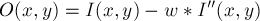### Convolution

```import gfxprim.core as core
import gfxprim.filters as filters

# Does in-place bilinear convolution with 3x3 box blur kernel
filters.Convolution(img, img, [[1, 1, 1],
[1, 1, 1],
[1, 1, 1]], 9, callback=None)

# Does bilinear convolution with 3x3 box blur kernel.
#
# The image data from source starting at 20,20 of a size 250x250 are
# stored at 100,100 in res.
filters.ConvolutionEx(img, 20, 20, 250, 250, res, 100, 100,
[[1, 1, 1],
[1, 1, 1],
[1, 1, 1]], 9, callback=None)

# Returns newly allocated image convolution with Laplacian 3x3 kernel
res = img.filters.ConvolutionAlloc([[ 0.00, -0.25,  0.00],
[-0.25,  1.00, -0.25],
[ 0.00, -0.25,  0.00]],
1, callback=None)

# Returns newly allocated subimage convolution with Sobel 3x3 kernel
res = img.filters.ConvolutionExAlloc(50, 50, 100, 100,
[[ 1, 0, -1],
[ 2, 0, -2],
[ 1, 0, -1]], 1, callback=None)```

Bilinear convolution. The kernel is specified as two dimensional array of numbers, the second number is divisor of the kernel weighed sum of pixels.

The pixel value is computed as: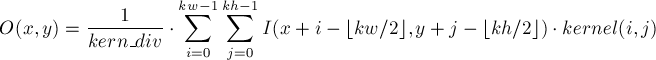Which is the same as: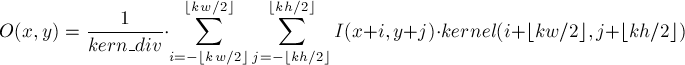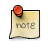The number of kernel rows and columns is expected to be odd number.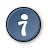See convolution example.

### Blurs

```import gfxprim.core as core
import gfxprim.filters as filters

# Does in-place Gaussian blur, the image is modified in-place
filters.GaussianBlur(img, img, x_sigma, y_sigma, callback=None)

# Returns newly alocated blurred image
res = img.filters.GaussianBlur(x_sigma, y_sigma, callback=None)
```

Gaussian blur (low pass) filters implemented as bilinear separable convolution.See blur example.
Original Image; Gaussian Blur xsig=2 ysig=2, xsig=0 ysig=4, xsig=4 ysig=0, xsig=4 ysig=4, xsig=10 ysig=10

### Ditherings

```import gfxprim.core as core
import gfxprim.filters as filters

# Returns img dithered to 1-bit Grayscale as a new image
res = img.filters.FloydSteinbergAlloc(core.C.PIXEL_G1, callback=None)

# Returns img dithered to 1-bit Grayscale as a new image
res = img.filters.HilbertPeanoAlloc(core.C.PIXEL_G1, callback=None)
```

Returns new 1-bit Grayscale image which is result from Floyd-Steinberg, Hilbert-Peano dithering.

The first parameter is pixel type, the second is progress callback.See dithering example.

### Median

```import gfxprim.core as core
import gfxprim.filters as filters

# Returns result of median filter over a rectangle of a side 2 * 3 + 1 pixels
res = img.filters.MedianAlloc(3, 3, callback=None)

# Applies median filter in-place
img.filters.Median(3, 3, callback=None)```

Constant time median filter (the computational complexity is independent of radius size).

The parameters are radius values for x and y. The algorithm uses x respectively y pixel neighbors from each side so the result is median of rectangle of `2 * x + 1` x `2 * y + 1` pixels.

Original Image; Median xr=3 yr=3, xr=5 yr=5, xr=7 yr=7, xr=9 yr=9, xr=12 yr=12

### Resize

```import gfxprim.core as core
import gfxprim.filters as filters

# Nearest neighbour resize, fastest but lowest quality
res = img.ResizeNNAlloc(100, 100, callback=None)

# Fast and good quality with low pass on downscaling
res = img.ResizeLinearLFIntAlloc(100, 100, callback=None)

# Cubic interpolation, needs low pass (blur) applied before downscaling
res = img.ResizeCubicIntAlloc(100, 100, callback=None)

# All of the above, TYPE is numeric enum
res = img.ResizeAlloc(100, 100, TYPE, callback=None)
```

Functions to resize (resample) image.See resize example.